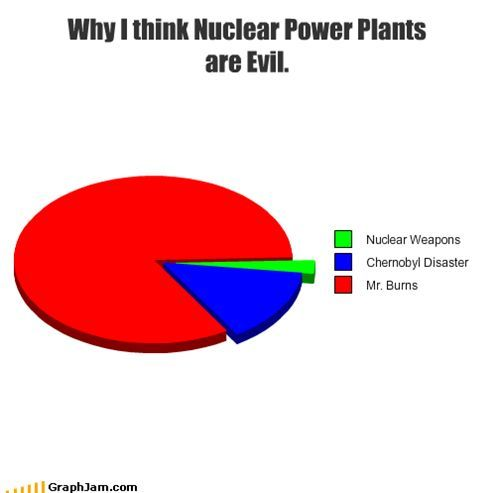Nuclear Chemistry Practice Test
3 years ago
lspencer42
Save
Edit
Host a game
Live GameLive
Homework
Solo Practice
Practice25 QuestionsShow answers
• Question 1
30 seconds
Q. What type of decay changes the atomic number of the atom?
Transmutation
Translation
Translocation
Transuranium
• Question 2
30 seconds
Q. Which of the following best describes the process of nuclear fission?
Splitting an atom's nucleus apart
Separating an atom's electrons from its nucleus
Fusing two atomic nuclei together
Splitting an atom's electrons in half
• Question 3
30 seconds
Q. Which of the following is an example of nuclear fusion?
A plutonium atom is used to start a chain reaction that detonates a nuclear weapon
A uranium atom is split apart into lighter elements
Two hydrogen atoms are combined to form a helium atom
Two hydrogen atoms bond with an oxygen atom to form a water molecule
• Question 4
30 secondsQ. Positively charged particles that consist of two protons and two neutrons are called
alpha particles
beta particles
gamma rays
neutron emissions
• Question 5
30 secondsQ. An electron that is emitted during radioactive decay is a
neutron
gamma ray
alpha particle
beta particle
• Question 6
30 seconds
Q. The least penetrating type of radiation
Alpha particle
Beta particle
gamma ray
• Question 7
30 seconds
Q. This type of reaction occurs on the sun and in stars.
Fission
Fusion
synthesis
decomposition
• Question 8
30 seconds
Q. A form of radiation that carries energy and has no mass and no charge.
Alpha particles
Beta Particles
Gamma Rays
Daughter nucleus
• Question 9
30 secondsQ. Which type of nuclear radiation is being emitted here?
alpha
beta
gamma
none
• Question 10
30 secondsQ. What element is the ?
Ag-115
Cd-115
Pd-115
Not Listed
• Question 11
30 seconds
Q. What type of decay does not change the element or the mass?
Alpha
Beta
Gamma
• Question 12
60 secondsQ. Which type of nuclear radiation is being emitted here?
Alpha
beta
gamma
none
• Question 13
60 seconds
Q. What is meant by the statement that 15P32 has a half life of 14 days
It takes 7 days to go down to 50% radioactivity each time
It takes 14 days to go down to 50% radioactivity each time
After 14 days it starts to become less radioactive
It takes 14 days to go up by 50% radioactivity each time
• Question 14
30 seconds
Q. The type of radioactive particle will penetrate farthest into a material is a(n)__________.
alpha
beta
gamma
all of the above
• Question 15
120 seconds
Q. How many neutrons does uranium-239 have
92
147
239
331
• Question 16
120 seconds
Q. What is Half-life?
The amount of time it takes for some of the nuclei in a sample of the isotope to decay
The amount of time it takes for half the electrons in a sample of the isotope to decay
The amount of time it takes for half the nuclei in a sample of the isotope to decay
the amount of time it takes to double the nuclei in a sample of the isotope to decay
• Question 17
900 seconds
Q. The half-life of Zn-71 is 2.4 minutes. If one had 100.0 g at the beginning, how many grams would be left after 7.2 minutes has elapsed?
100.0g
50.0g
12.5g
8.5g
• Question 18
900 seconds
Q. Barium-122 has a half-life of 2 minutes. A fresh sample weighing 80 g was obtained. If it takes 10 minutes to set up an experiment using barium-122, how much barium-122 will be left when the experiment begins?
0.25g
2.5g
25g
80g
• Question 19
120 seconds
Q. What is the symbol for an alpha particle?
42He
0-1e
0+1 e
10n
• Question 20
120 seconds
Q. In a nuclear reactor what slows down neutrons?
Control Rods
Moderator
Sheilding
The brakes
• Question 21
120 seconds
Q. In a nuclear reactor what absorbs fast moving neutrons?
generator
moderator
control rods
sheilding
• Question 22
120 seconds
Q. In fission neutron is often used to start the reaction and it is also a product of the reaction. This is called a ____
neutron emission
nuclear fusion
domino effect
chain reaction
• Question 23
30 seconds
Q. What turns the turbines in a nuclear power plant?
uranium
plutonium
heat
steam
• Question 24
30 seconds
Q. What type of nuclear decay emits a positive electron from the nucleus of an atom?
beta decay
gamma decay
alpha decay
positron emission
• Question 25
30 seconds
Q. Which of the following is NOT a use for radiation?